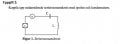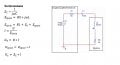# Theoretical values for ”natural frequency”, currents and voltage at electrical resonance.

Joined Feb 11, 2019
22
I am supposed to calculate the theoretical values for ”natural frequency”, currents and voltages at electrical resonance for this circuit.

I should use the measured values for the resistance and the inductance of the coil. Also the measured value of the capacitance to the capacitor. The internal resistance of signal generator must be used in the calculations!

The voltage source should be used as a references for all phase angles.Measured Values:
Inductance of a Coil:
56,91 mH
Theoretical Resonance: 980 Hz
Resistance of the Coil: 36 Ω
Capacitor: 481,7 uF
U: 7,02 V @ 1KHz

"Spole" = Coil#### WBahn

Joined Mar 31, 2012
28,185

What conditions do you think apply at the natural frequency of the circuit?

Joined Feb 11, 2019
22

What conditions do you think apply at the natural frequency of the circuit?
Okay, i am reading on the subject right now. I don't know the answear to your question.

#### WBahn

Joined Mar 31, 2012
28,185
Okay, i am reading on the subject right now. I don't know the answear to your question.
So that should be a focus of your reading -- namely to find out what, exactly, it means for a particular frequency to be the "natural" frequency of a circuit.

#### MrAl

Joined Jun 17, 2014
9,780
I am supposed to calculate the theoretical values for ”natural frequency”, currents and voltages at electrical resonance for this circuit.

I should use the measured values for the resistance and the inductance of the coil. Also the measured value of the capacitance to the capacitor. The internal resistance of signal generator must be used in the calculations!

The voltage source should be used as a references for all phase angles.

View attachment 197408

Measured Values:
Inductance of a Coil:
56,91 mH
Theoretical Resonance: 980 Hz
Resistance of the Coil: 36 Ω
Capacitor: 481,7 uF
U: 7,02 V @ 1KHz